# 跟我学 Python 图像处理丨傅里叶变换之高通滤波和低通滤波

• 2022 年 9 月 28 日
中国香港
• 本文字数：2017 字

阅读完需：约 7 分钟

# 一.高通滤波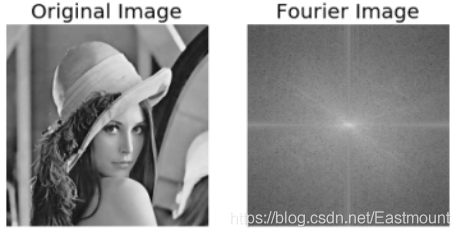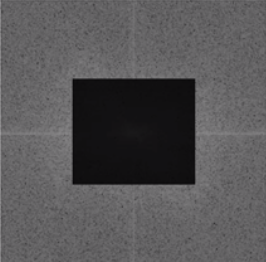rows, cols = img.shapecrow,ccol = int(rows/2), int(cols/2)fshift[crow-30:crow+30, ccol-30:ccol+30] = 0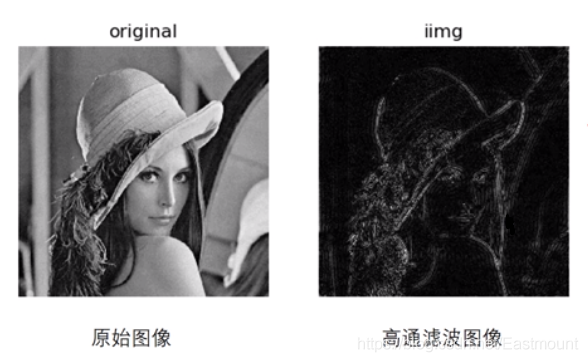# -*- coding: utf-8 -*-import cv2 as cvimport numpy as npfrom matplotlib import pyplot as plt#读取图像img = cv.imread('Lena.png', 0)#傅里叶变换f = np.fft.fft2(img)fshift = np.fft.fftshift(f)#设置高通滤波器rows, cols = img.shapecrow,ccol = int(rows/2), int(cols/2)fshift[crow-30:crow+30, ccol-30:ccol+30] = 0#傅里叶逆变换ishift = np.fft.ifftshift(fshift)iimg = np.fft.ifft2(ishift)iimg = np.abs(iimg)#显示原始图像和高通滤波处理图像plt.subplot(121), plt.imshow(img, 'gray'), plt.title('Original Image')plt.axis('off')plt.subplot(122), plt.imshow(iimg, 'gray'), plt.title('Result Image')plt.axis('off')plt.show()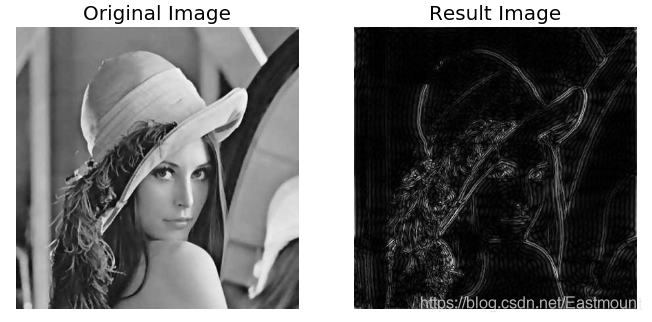# 二.低通滤波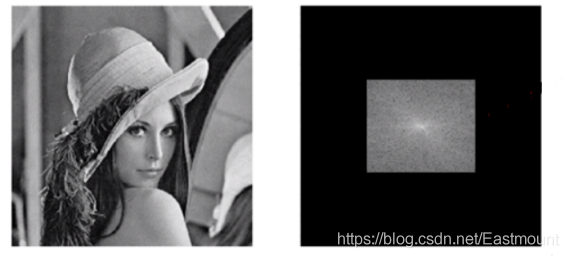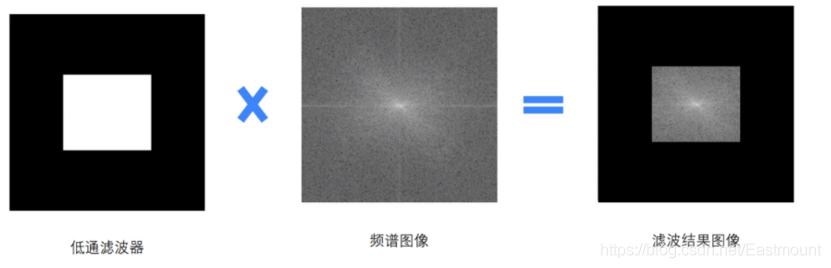rows, cols = img.shapecrow,ccol = int(rows/2), int(cols/2)mask = np.zeros((rows, cols, 2), np.uint8)mask[crow-30:crow+30, ccol-30:ccol+30] = 1

# -*- coding: utf-8 -*-import cv2import numpy as npfrom matplotlib import pyplot as plt#读取图像img = cv2.imread('lena.bmp', 0)#傅里叶变换dft = cv2.dft(np.float32(img), flags = cv2.DFT_COMPLEX_OUTPUT)fshift = np.fft.fftshift(dft)#设置低通滤波器rows, cols = img.shapecrow,ccol = int(rows/2), int(cols/2) #中心位置mask = np.zeros((rows, cols, 2), np.uint8)mask[crow-30:crow+30, ccol-30:ccol+30] = 1#掩膜图像和频谱图像乘积f = fshift * maskprint f.shape, fshift.shape, mask.shape#傅里叶逆变换ishift = np.fft.ifftshift(f)iimg = cv2.idft(ishift)res = cv2.magnitude(iimg[:,:,0], iimg[:,:,1])#显示原始图像和低通滤波处理图像plt.subplot(121), plt.imshow(img, 'gray'), plt.title('Original Image')plt.axis('off')plt.subplot(122), plt.imshow(res, 'gray'), plt.title('Result Image')plt.axis('off')plt.show()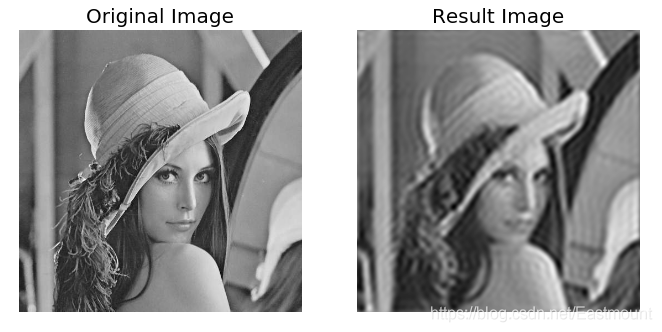• 《数字图像处理》（第 3 版），冈萨雷斯著，阮秋琦译，电子工业出版社，2013 年.

• 《数字图像处理学》（第 3 版），阮秋琦，电子工业出版社，2008 年，北京.

• 《OpenCV3 编程入门》，毛星云，冷雪飞，电子工业出版社，2015，北京.

• 百度百科-傅里叶变换

• 网易云课堂-高登教育 Python+OpenCV 图像处理## 评论